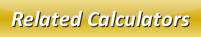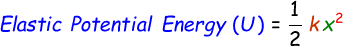# Elastic Potential Energy Calculator

The elastic potential energy is a type of potential energy that is stored within an elastic material such as spring, rubber band, bow etc.

Formula to calculate elastic potential energy is given below:where,
U = Elastic potential energy [J]
k = elastic force constant [N/m]
x = stretch length [m]

Use our below online elastic potential energy calculator to find the potential energy stored in the elastic object. Enter the elastic force constant and stretch length and click calculate to find the elastic potential energy.

 Elastic Force Constant (k): [N/m] Stretch Length (x): [m] Elastic Potential Energy (U): [J]

Latest Calculator Release

Average Acceleration Calculator

Average acceleration is the object's change in speed for a specific given time period. ...

Free Fall Calculator

When an object falls into the ground due to planet's own gravitational force is known a...

Torque Calculator

Torque is nothing but a rotational force. In other words, the amount of force applied t...

Average Force Calculator

Average force can be explained as the amount of force exerted by the body moving at giv...

Angular Displacement Calculator

Angular displacement is the angle at which an object moves on a circular path. It is de...